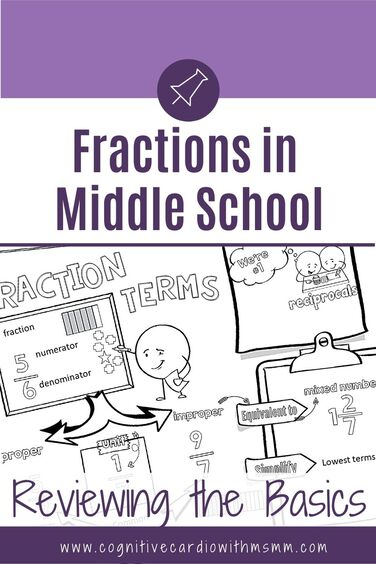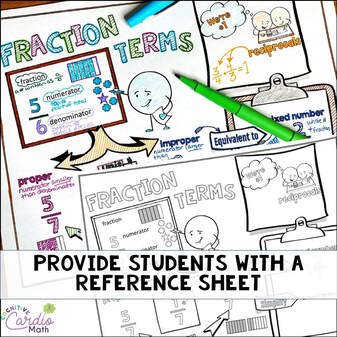# Teaching Fractions in Middle School: Reviewing the BasicsWelcome to this blog series about teaching fractions in middle school!
For the next several weeks, we’ll be looking at various aspects of fractions: basic meanings, fraction models, finding equivalent fractions, comparing fractions, benchmarks and estimation.

As an upper elementary or middle school math teacher, you may find yourself needing to review some of these fraction concepts before you can move on to the fraction curriculum for your grade level.
When this is the case (needing to review fraction concepts that aren’t part of your curriculum), there usually isn’t much time to squeeze in extra instruction…it can be tough!
We realize that some of our middle school kids just don’t ‘get’ fractions, but our time is limited. I’ve got a couple ideas at the end of the post for how to weave in some of the ‘fraction basics’ in middle school.

But first, for this week, we’ll start with some of the foundational fraction vocabulary terms.

## Fraction Terms

First up ~ fraction, numerator and denominator. These are the easy ones, right?
We might think so, but sometimes students have misunderstandings around these terms. When I looked for definitions of these terms, I found the following:

• Fraction: “a number, written in the form a/b” and “a part of a whole, less than one”
• Numerator: “the top number in a fraction that tells how many parts are being considered” or “the number above the line in a common fraction, showing how many of the parts indicated by the denominator are taken”
• Denominator: “the bottom number that tells how many equal parts in a whole,” “the number below the line in a common fraction,” and “a divisor”

When students learn about fractions in the earlier grades, fractions are represented with images like those below, with the numerator being the colored part (or white part) and the denominator being the total number of pieces.

In 3rd grade, if the class is following common core standards, students should also learn that a fraction is a number on the number line.

• Using the number line and the definition of “a number written in the form a/b” helps reinforce the idea that a fraction is a number that represents an amount, just as the number 2 represents two whole things.
By middle school, it often seems that the picture with colored/not colored sections is what students remember best. Not the number line. Do you find this too?

The number line model is so important when students start learning about decimals on the number line (and it can help them understand equivalent fractions), so if your middle schoolers aren’t familiar with fractions on the number line, I’d recommend making this a big focus.

• When students understand that both fractions and decimals can be represented in the same way on the number line, it helps their understanding of the equivalence of decimals and fractions (and later percents). Fractions, decimals, and percents all represent parts of a whole…they’re just written differently.

​We’ll look at fraction models more in depth in a future post.

A few other important terms we’ll explore in the coming weeks:
Equivalent fractions:

• Fractions that have the same value, or represent the same amounts.
• This can be modeled as 2 shapes of the same size, cut into different numbers of pieces.
• This can be modeled as two fractions marked by the same place on a number line (or ruler) that show the same number.

Improper fractions: fractions that represent an amount greater than 1, so the numerator is larger than the denominator (I know improper fractions are now often referred to as ‘fractions greater than one’ but I think it’s important for students to know and understand this term…..it’s still used in standardized tests at different levels
Mixed numbers: also represent amounts greater than 1, and are the combination of a whole number and a proper fraction
Unit fractions: fractions with a numerator of one

## Daily Fraction ReinforcementMiddle students often come to us with varied fraction backgrounds, and as mentioned, some students don’t seem to understand what fractions even are.

I’ve found that weaving fractions into parts of our math routine helps keep fraction basics fresh. It also helps students be more prepared for when they must use fractions and fraction operations in other math contexts. A few ideas include:

1) Review fraction vocabulary at the beginning of the year and provide students with a type of reference sheet.
(The fraction doodle page shown here is available in the free resource center on this site. To access this and other free resources, check out the Free
Resource Center Information page.)
​​

2) Keep visual representations around the room. For years, I kept a fraction/decimal/percent number line up above my whiteboard. This kept some of the common fractions and their decimal/percent equivalences visible at all times for quick reference. Students definitely used this.
​Visuals of fraction sets, number lines, and partitioned shapes are all helpful.

3) Include fraction basics in spiral review. If the concepts keep popping up when you aren’t ‘teaching’ them, students will become more accustomed to seeing them and working with them.

4) If you do Number Talks, include fraction number talks as often as you’re able. These will give students a chance to explore fraction concepts a bit. If you don’t do Number Talks, consider checking them out!

• Quick example: you can do a Number Talk where you ask students to consider whether 3/8 is more or less than ½.

What other suggestions do you have to help middle school math students with the fraction basics?

Interested in more fraction and fraction operation content?
Check out the course,

## Fraction Operations Wheel

 Download this free fraction operations math wheel (plus other free fraction goodies) when you join the Cognitive Cardio Math community:-)

## EllieWelcome to Cognitive Cardio Math! I’m Ellie, a wife, mom, grandma, and dog ‘mom,’ and I’ve spent just about my whole life in school! With nearly 30 years in education, I’ve taught:

• All subject areas in 4th and 5th grades
• Math, ELA, and science in 6th grade (middle school)

I’ve been creating resources for teachers since 2012 and have worked in the elearning industry for about five years as well!